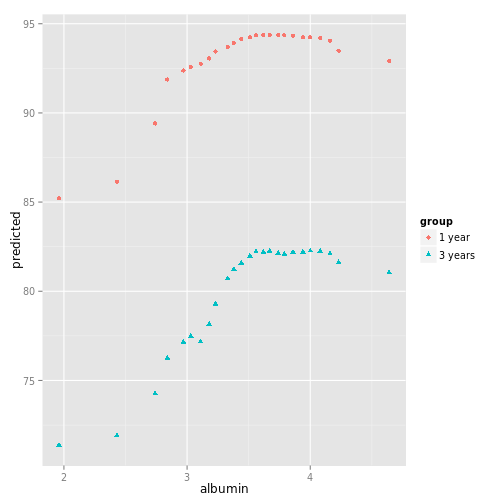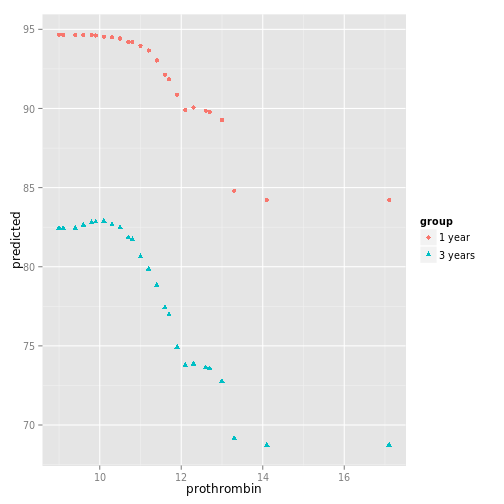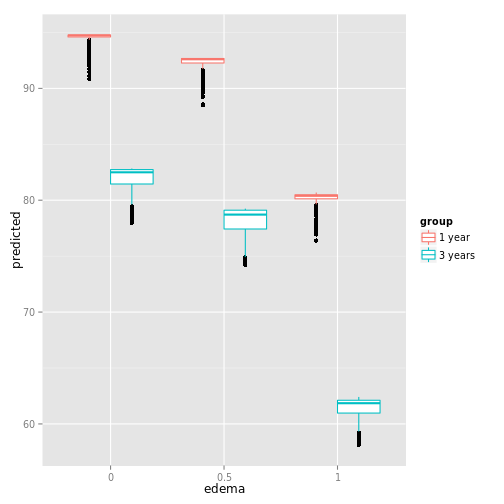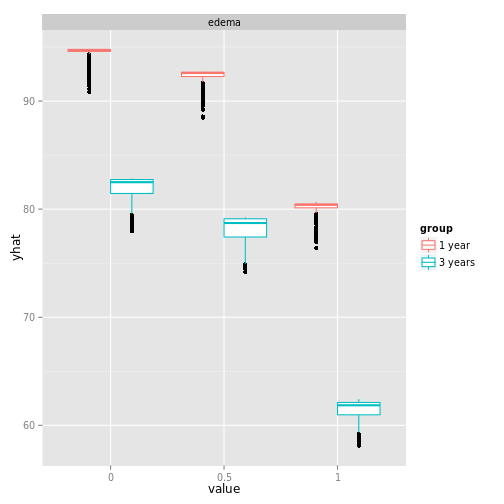# combine two gg_partial objects

``````# Load a set of plot.variable partial plot data
data(partial_pbc)

# A list of 2 plot.variable objects
length(partial_pbc)``````
``##  3``
``class(partial_pbc)``
``##  "list"``
``class(partial_pbc[])``
``##  "rfsrc"         "plot.variable" "surv"``
``class(partial_pbc[])``
``##  "rfsrc"         "plot.variable" "surv"``
``````# Create gg_partial objects
ggPrtl.1 <- gg_partial(partial_pbc[])
ggPrtl.2 <- gg_partial(partial_pbc[])

# Combine the objects to get multiple time curves
# along variables on a single figure.
ggpart <- combine.gg_partial(ggPrtl.1, ggPrtl.2,
lbls = c("1 year", "3 years"))

# Plot each figure separately
plot(ggpart)``````
``## []````````##
## []````````````##
## []````````````##
## []````````````##
## []````````````##
## []````````````# Get the continuous data for a panel of continuous plots.
ggcont <- ggpart
ggcont\$edema <- ggcont\$ascites <- ggcont\$stage <- NULL
plot(ggcont, panel=TRUE)``````
``````## geom_smooth: method="auto" and size of largest group is <1000, so using loess. Use 'method = x' to change the smoothing method.
## geom_smooth: method="auto" and size of largest group is <1000, so using loess. Use 'method = x' to change the smoothing method.
## geom_smooth: method="auto" and size of largest group is <1000, so using loess. Use 'method = x' to change the smoothing method.
## geom_smooth: method="auto" and size of largest group is <1000, so using loess. Use 'method = x' to change the smoothing method.
## geom_smooth: method="auto" and size of largest group is <1000, so using loess. Use 'method = x' to change the smoothing method.````````````# And the categorical for a panel of categorical plots.
nms <- colnames(sapply(ggcont, function(st){st}))
for(ind in nms){
ggpart[[ind]] <- NULL
}
plot(ggpart, panel=TRUE)``````Updated